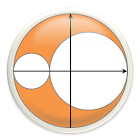All Android applications categories

All Android games categoriesby: 20 9

9 Users
rating

## Screenshots

Description

This Mohr's Circle calculator for Android makes it easy to generate 2D and 3D Mohr's Circle for both stresses and strains.

Use on the job! Check your homework! Have fun!

Features:
- 2D Mohr's Circle.
- 3D Mohr's Circle.
- Strain rosette input with *any* gauge angles (new!).
- Convert between stress and strain, & 2D and 3D.
- Compute principal stresses and principal strains.
- Compute maximum shear stresses and strains.
- Determine the shear modulus (G), bulk modulus (K), and Lame's 1st constant from Young's modulus and Poisson's ratio.
- View tensor properties such as principal directions and von Mises stress.

Tags: mohrs circle calculator , mohr circle calculator , 3d mohr circle calculator , mohr's circle calculator , mohrs circle , 2d mohrs circle calculator , gambar 3d principal stress , mohrs circle strain rosette calculator , mohr tool circle

from 20 reviews

"Awesome"

9